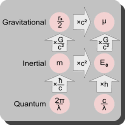Friday , 27 May 2022

# What is the Schwarzschild Radius?The Schwarzschild Radius is a characteristic radius associated with every quantity of mass. It is a radius of a sphere in space, that if containing a corresponding sufficient amount of mass, the force of gravity from the contained mass would be so great that no known force or pressure could stop the mass from continuing to collapse in volume into a point of infinite density: a gravitational singularity. This term is used in physics and astronomy, especially in the theory of gravitation and general relativity. In 1916, Karl Schwarzschild obtained an exact solution to Einstein’s field equations for gravitational field outside a non-rating, spherically symmetric body. The solution contained a formula, where one of the values came to be known as Schwarzschild Radius.

## Jawahar Lal Nehru Death Anniversary Information

This year will mark death anniversary of country’s first Prime Minister Jawahar Lal Nehru on …